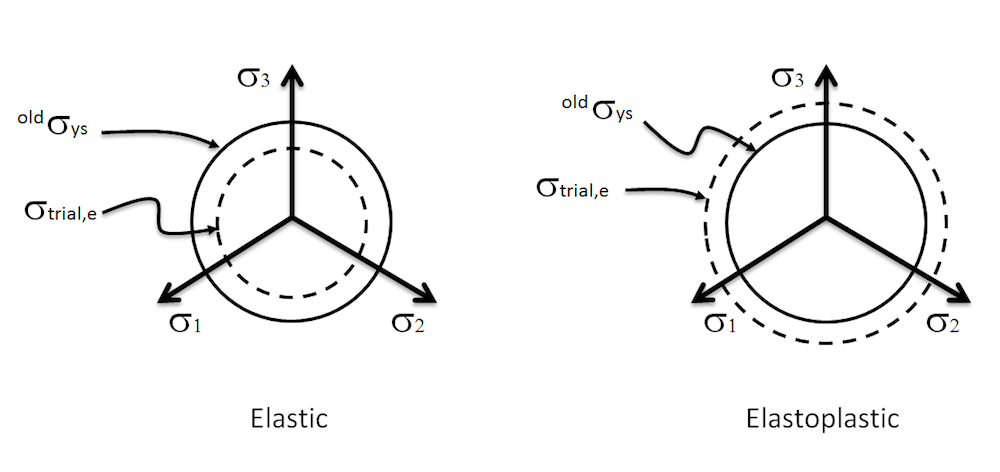# 如何调用外部材料在模型中实现弹塑性

2017年 11月 2日

### 外部材料特征

COMSOL Multiphysics® 的附加产品——非线性结构材料模块内置了大量的材料模型，包括超弹性、各向同性/运动硬化塑性、黏弹性、蠕变、多孔塑性、土壤等模型。这些材料模型涵盖了结构分析中的绝大多数工程问题。

1. 许多材料模型都有用户定义 选项。例如，你可以通过在 GUI 中直接输入应变能量密度与应变函数的表达式来创建自己的超弹性材料。
2. 下一个级别，当没有合适的材料模型可以通过用户定义 选项调整时，可以将材料模型表示为一组单独的 PDE 或 ODE，并使用其中一个数学接口输入适当的表达式。
3. 最后，你可以使用外部材料特征 用 C 代码编写自己的材料模型。如果材料模型表示为一种算法，而不是一组方程，这是首选方法。

### 在 COMSOL Multiphysics® 中实现弹塑性材料模型

• 应变是相加的，假设很小
• 弹性响应是线性和各向同性的，由杨氏模量 E 和泊松比 ν 定义（或等效地由体积和剪切模量 KG 定义）
• 塑性响应是各向同性的，初始屈服应力为 \sigma_{ys0}
• 材料的屈服应力是有效塑性应变的函数，\sigma_{ys}=\sigma_{ys0}
+\sigma_h\left(\epsilon_{pe}\right)
，具有初始值 \sigma_{h}\left(0\right)=0 (硬化函数)
• 塑性流动遵循 J_2 理论
• 有效塑性应变的增量由下式给出 \Delta\epsilon_{pe}=\left(\frac{2}{3}\Delta\bm\epsilon_p:\Delta\bm\epsilon_p\right)^{1/2}#### 方法 1：广义应力-应变关系

1. 应力 张量 \bm{\sigma} 的计算
2. 应力 \bm{\sigma} 相对于应变 \bm{\epsilon} 的雅可比行列式的计算

#### 方法 2：非弹性残余应变

1. 非弹性应变 张量的计算 \bm {\epsilon}_{inel}
2. 非弹性应变 \bm {\epsilon}_{inel} 相对于应变的 \bm {\epsilon} 雅可比行列式的计算

### 应力张量的计算：应力更新算法

• 对应于当前位移场估计的总应变
• 之前收敛增量的材料状态

\mathrm{dev}\left(\bm\sigma_{trial}\right) = 2G\,\mathrm{dev}\left(\bm\epsilon-^{old}\bm\epsilon_{p}\right)

^{old}\sigma_{ys} = \sigma_{ys0}+\sigma_h\left(^{old}\epsilon_{pe}\right)

f=\left\{\begin{array}{c l}\sigma_{trial,e}-^{old}\sigma_{ys}<0 & \mathrm{Elastic}\\ \sigma_{trial,e}-^{old}\sigma_{ys}\ge0 & \mathrm{Elastoplastic}\end{array}\right.#### 弹性计算

\left\{\begin{array}{lcl}\bm\sigma &=& \mathrm{dev}\left(\bm\sigma_{trial}\right) + K\mathrm{trace}\left(\bm\epsilon\right)\mathbf{1}\\\bm\epsilon_p &=& ^{old}\bm\epsilon_p\\\epsilon_{pe} &=& ^{old}\epsilon_{pe}\end{array}\right.

#### 弹塑性计算

\mathrm{dev}\left(\bm\sigma\right) =2G\,\mathrm{dev}\left(\bm\epsilon-^{old}\bm\epsilon_{p}-\Delta\bm\epsilon_p\right)=\mathrm{dev}\left(\bm\sigma_{trial}\right)- 2G\Delta{\lambda}\,\mathrm{dev}\left(\bm{\sigma}\right)

\mathrm{dev}\left(\bm\sigma\right) = \frac{1}{\left(1+2G\Delta\lambda\right)}\mathrm{dev}\left(\bm\sigma_{trial}\right)1. 将应力方程转换为控制标量方程
2. 在单个参数中表达所有未知数
3. 求解该参数的标量方程

\sigma_{ys0}+\sigma_h\left(^{old}\epsilon_{pe} + \Delta\epsilon_{pe}\right)+3G\Delta\epsilon_{pe}-\sigma_{trial,e}=0.

\left\{ \begin{array}{ccl}\bm\sigma &=& \frac{1}{1+2G\Delta\lambda}\mathrm{dev}\left(\bm\sigma_{trial}\right) + K\mathrm{trace}\left(\bm\epsilon\right)\mathbf{1}\\ \\\bm\epsilon_p &=& ^{old}\bm\epsilon_p + \Delta\bm\epsilon_p\\\epsilon_{pe} &=& ^{old}\epsilon_{pe} + \Delta\epsilon_{pe} \end{array}\right.

### 计算材料的雅可比行列式

\begin{array}{rcl} \mathrm{dev}\left(\bm\sigma\right) &=& \mathrm{dev}\left(\bm\sigma_{trial}\right)-2G\,\Delta\bm\epsilon_p \\\mathrm{trace}\left(\bm\sigma\right)&=&3K\mathrm{trace}\left(\bm\epsilon\right)\end{array}.

\mathrm{d}\bm\sigma=\mathcal{C}:\mathrm{d}\bm\epsilon

\mathrm{d}\bm\sigma= \underbrace{ \left[ \underbrace{2G\left(\mathcal{I}-\frac{1}{3}\mathbf{1}\otimes\mathbf{1}\right) + K\left(\mathbf{1}\otimes\mathbf{1}\right)}_{\mathcal{C}^{e}} – 2G\frac{\mathrm{d}\bm\epsilon_p}{\mathrm{d}\bm\epsilon} \right]}{\mathcal{C}}:\mathrm{d}\bm{\epsilon}

\frac{ \mathrm{d}\bm\epsilon{inel}}{\mathrm{d}\epsilon}=\frac{\mathrm{d}\bm\epsilon_p}{\mathrm{d}\epsilon}

\mathcal{C}^e 是弹性雅可比行列式。对于纯弹性计算，广义应力应变 关系的总雅可比等于该量，这时非弹性残余应变 的雅可比为零。

\frac{\mathrm{d}\bm\epsilon_p}{\mathrm{d}\bm\epsilon} = \Delta\lambda\frac{\mathrm{d}\,\mathrm{dev}\left(\bm\sigma\right)}{\mathrm{d}\bm\epsilon}\,+ \,\mathrm{dev}\bm\sigma\otimes\frac{\mathrm{d}\Delta\lambda}{\mathrm{d}\bm\epsilon}

\frac{\mathrm{d}\Delta\lambda}{\mathrm{d}\bm\epsilon} = \frac{9G}{2\sigma_{ys}^2} \, \frac{1}{3G+\frac{\mathrm{d}\sigma_h}{\mathrm{d}\Delta\epsilon_{pe}}} \, \left(1-\frac{2} {3}\Delta\lambda\frac{\mathrm{d}\sigma_h}{\mathrm{d}
\Delta\epsilon_{pe}}\right) \, \mathrm{dev}
\left(\sigma\right)

#### 关于雅可比计算的进一步评论

• 外部材料对应力、应变和雅可比行列式使用简化的矢量和矩阵形式。我们需要相应地表述应力更新算法和雅可比计算。
• 进入外部材料的应变矢量使用张量分量表示剪切力。雅可比行列式的定义是假设剪切的张量分量；因此，它通常是非对称的。
• 平面应力是在外部材料特征之外处理的，所以我们只需要对模型进行一次实现。换句话说，我们实现的模型可以直接用于 3D、2D 轴对称和 2D 平面应力和平面应变分析。

### 应用示例：材料模型的专业化

\Delta\epsilon_{pe}= \frac{\sigma_{trial,e}-^{old}\sigma_{ys}}{3G+E_{iso}}• E = 70 GPa
• n = 0.2
• \sigma_{ys0}= 243 MPa
• E_{Tiso} = 2.17 Pa

### 下一步

• 下载本博客中的示例源代码和模型文件(linear_hardening.mph, linear_hardening.c, linear_hardening.dll) 并获得更多关于如何使用外部材料的详细信息:
• 阅读之前的博客文章：在结构力学仿真中访问外部材料模型
• 阅读以下书籍，了解更多关于塑性计算的信息:
• J.C. Simo and T.J.R. Hughes, Computational Inelasticity, Interdisciplinary Applied Mathematics, Vol. 7, Springer-Verlag, 1998.
• M. Kojic and K.J. Bathe, Inelastic Analysis of Solids and Structures, Computational Fluid and Solid Mechanics, Springer-Verlag, 2005.
• T. Belytschko, W.K. Liu, and B. Moran, Nonlinear Finite Elements for Continua and Structures, Wiley, 2000.

PolyUMod 软件是由 Veryst Engineering LLC 开发的。COMSOL AB 及其子公司和产品与 Veryst Engineering LLC 没有关系，也没有得到 Veryst Engineering LLC 的认可、赞助或支持。